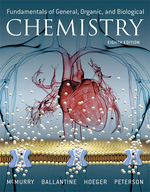×
Get Full Access to Fundamentals Of General, Organic, And Biological Chemistry (Mastering Chemistry) - 8 Edition - Chapter 5 - Problem 5.62
Get Full Access to Fundamentals Of General, Organic, And Biological Chemistry (Mastering Chemistry) - 8 Edition - Chapter 5 - Problem 5.62

×ISBN: 9780134015187 2044

## Solution for problem 5.62 Chapter 5

Fundamentals of General, Organic, and Biological Chemistry (Mastering Chemistry) | 8th Edition

• Textbook Solutions
• 2901 Step-by-step solutions solved by professors and subject experts
• Get 24/7 help from StudySoup virtual teaching assistantsFundamentals of General, Organic, and Biological Chemistry (Mastering Chemistry) | 8th Edition

4 5 1 260 Reviews
22
1
Problem 5.62

Balance the following equations and classify each as a precipitation, neutralization, or redox reaction:

(a) $$\begin{array}{l} \mathrm{Al}(\mathrm{OH})_{3}(a q)+\mathrm{HNO}_{3}(a q) \longrightarrow\\ \mathrm{Al}\left(\mathrm{NO}_{3}\right)_{3}(a q)+\mathrm{H}_{2} \mathrm{O}(l) \end{array} ) (b) \(\mathrm{AgNO}_{3}(a q)+\mathrm{FeCl}_{3}(a q) \underset{\mathrm{AgCl}(s)+\mathrm{Fe}\left(\mathrm{NO}_{3}\right)_{3}(a q)}{\longrightarrow}$$

(c) $$\left(\mathrm{NH}_{4}\right)_{2} \mathrm{Cr}_{2} \mathrm{O}_{7}(s) \longrightarrow \mathrm{Cr}_{2} \mathrm{O}_{3}(s)+\mathrm{H}_{2} \mathrm{O}(g)+\mathrm{N}_{2}(g)$$

(d) $$\mathrm{Mn}_{2}\left(\mathrm{CO}_{3}\right)_{3}(s) \longrightarrow \mathrm{Mn}_{2} \mathrm{O}_{3}(s)+\mathrm{CO}_{2}(g)$$

Text Transcription:

AI (OH)_3 (Aq) + HNO_3 (aq) arrow right Ai (NO_3)_3 (aq) + H_2O(I)

AgNO_3 (aq) + FeCI_3 (Aq) arrow right AgCI (S) + Fe (NO_3)_3 (Aq)

(NH_4)_2Cr_2O_7 (s) arrow right Cr_2O_3 (s) + H_2O (g) + N_@ (g)

Mn_2 (CO_3)_3 (s) arrow right Mn_2O_3 (s) + CO_2 (g)

Step-by-Step Solution:
Step 1 of 3

Step 2 of 3

Step 3 of 3

##### ISBN: 9780134015187

Since the solution to 5.62 from 5 chapter was answered, more than 200 students have viewed the full step-by-step answer. This textbook survival guide was created for the textbook: Fundamentals of General, Organic, and Biological Chemistry (Mastering Chemistry), edition: 8. The answer to “?Balance the following equations and classify each as a precipitation, neutralization, or redox reaction: (a) $$\begin{array}{l}\mathrm{Al}(\mathrm{OH})_{3}(a q)+\mathrm{HNO}_{3}(a q) \longrightarrow\\\mathrm{Al}\left(\mathrm{NO}_{3}\right)_{3}(a q)+\mathrm{H}_{2} \mathrm{O}(l)\end{array})(b) \(\mathrm{AgNO}_{3}(a q)+\mathrm{FeCl}_{3}(a q) \underset{\mathrm{AgCl}(s)+\mathrm{Fe}\left(\mathrm{NO}_{3}\right)_{3}(a q)}{\longrightarrow}$$ (c) $$\left(\mathrm{NH}_{4}\right)_{2} \mathrm{Cr}_{2} \mathrm{O}_{7}(s) \longrightarrow \mathrm{Cr}_{2} \mathrm{O}_{3}(s)+\mathrm{H}_{2} \mathrm{O}(g)+\mathrm{N}_{2}(g)$$(d) $$\mathrm{Mn}_{2}\left(\mathrm{CO}_{3}\right)_{3}(s) \longrightarrow \mathrm{Mn}_{2} \mathrm{O}_{3}(s)+\mathrm{CO}_{2}(g)$$Text Transcription:AI (OH)_3 (Aq) + HNO_3 (aq) arrow right Ai (NO_3)_3 (aq) + H_2O(I)AgNO_3 (aq) + FeCI_3 (Aq) arrow right AgCI (S) + Fe (NO_3)_3 (Aq)(NH_4)_2Cr_2O_7 (s) arrow right Cr_2O_3 (s) + H_2O (g) + N_@ (g)Mn_2 (CO_3)_3 (s) arrow right Mn_2O_3 (s) + CO_2 (g)” is broken down into a number of easy to follow steps, and 83 words. The full step-by-step solution to problem: 5.62 from chapter: 5 was answered by Aimee Notetaker, our top Chemistry solution expert on 04/25/22, 03:57PM. Fundamentals of General, Organic, and Biological Chemistry (Mastering Chemistry) was written by Aimee Notetaker and is associated to the ISBN: 9780134015187. This full solution covers the following key subjects: . This expansive textbook survival guide covers 281 chapters, and 456 solutions.

## Discover and learn what students are asking

Statistics: Informed Decisions Using Data : Applications of the Normal Distribution
?Explain why P(X ? 220) should be reported as > 70.9999 if X is a normal random variable with mean 100 and standard deviation 15.

Statistics: Informed Decisions Using Data : Testing the Significance of the Least-Squares Regression Model
?In Problems 5–10, use the results of Problems 7–12, respectively, from Section 4.2 to answer the following questions: (a) What are the estima

Statistics: Informed Decisions Using Data : Inference about the Difference between Two Medians: Independent Samples
?In Problems 1–8, use the Mann–Whitney test to test the given hypotheses at the = 0.05 level of significance. The independent samples were obtained ra

Unlock Textbook Solution